×

Search anything:

# Perlin Noise (with implementation in Python)

#### Algorithms Machine Learning (ML)# Introduction

One of the most important algorithms in computer graphics and procedural generation is Perlin Noise. Perlin Noise is an algorithm that generates textures and terrain-like images procedurally (without the need for an artist to manually create the images). Ken Perlin is the creator of this algorithm, for which he also won an Academy Award in 1997.

### What is a noise function?

There are numerous natural objects in the environment, like mountains, clouds, terrains, etc. that have some randomness in their texture. This randomness gives them their nature-like appearance. To mimic this appearance, we use algorithms like Perlin Noise. Using a procedural generating system like Perlin to generate random numbers, it is easy to reproduce the appearance of objects found in nature. Noise functions in such cases help in generating "noise" i.e random numbers by generating random variations in computer-generated models.

### Fractals

Completely random noise or numbers that are completely random in the computer-generated model don't look that natural. This is because even though natural objects have randomness in their texture, they also have some structure. The objects present in the natural environment also have some patterns that repeat. The variations present in their texture and their intensity depends on the size of the scale they are viewed in. At different scales, we see different repeating patterns and these patterns are many times similar to each other at different scales. This is also known as self-similarity and the concept of Fractals deals with such self-similar patterns. In such cases, Perlin noise helps in generating pseudo-random variations. It generates numbers that appear random but aren't actually, which helps to give computer-generated models a more natural appearance.

# Algorithm

Let's create a Perlin noise graph in 2D. Consider a pixel point Z, which is a scalar value. We create a grid with many scaler values like Z, as shown below.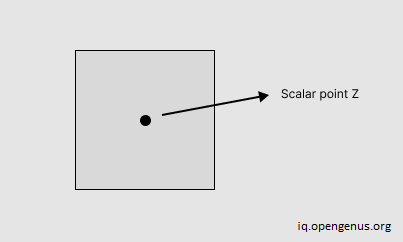Assume the image we'll generate is like a grid in the image below.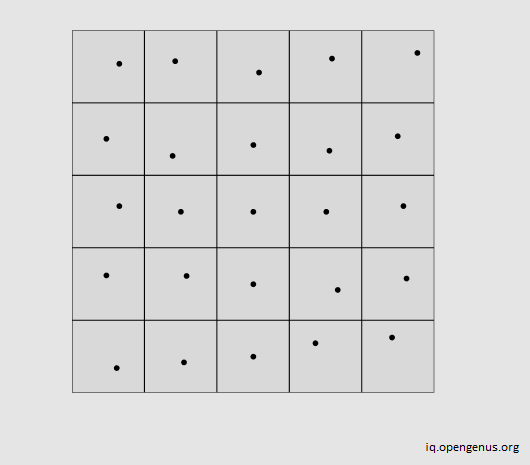Then for each of these points, we assign a random gradient vector. This vector is random in direction. In the image below, we can see that each point will have four gradient vectors in 2D. All gradient vectors in this case have a consistent length, usually of 1. So four gradient vectors will bound every pixel point like Z.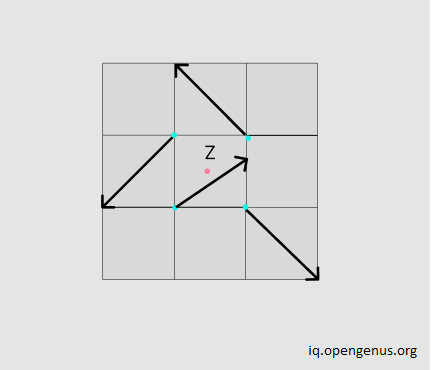Each gradient vector appears random but is in reality pseudorandom in nature and generates the same gradient value every time we calculate using the Perlin algorithm.

We calculate the distance vectors that start from the respective point on the grid to the subsequent gradient vectors surrounding it. To calculate this we subtract the point value of the gradient from the point on the grid.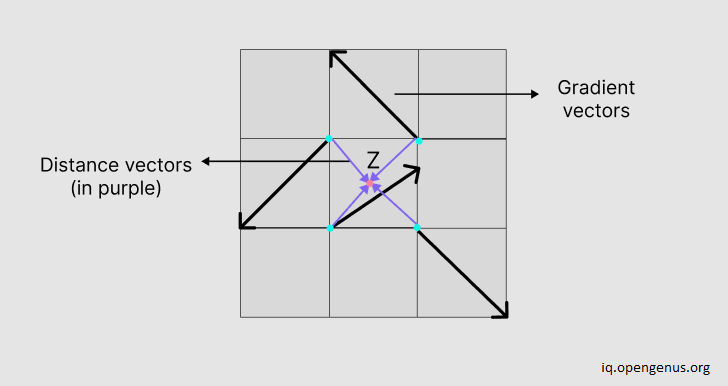The Perlin graph is almost done, but it won't be smooth in appearance. To do that, we apply the smooth function, given by the equation -

6t5 - 15t4 + 10t3

The first-order derivative and the second-order derivative both are zero for this function. This helps to give the generated image/model more smooth appearance over time.

After applying this function to the distance vector coordinates of each pixel inside the grid, we then calculate the gradient vectors. In the end, we use linear interpolation, which is just the dot product of the gradient vectors with the distance vectors.

Use the following code to generate a Perlin noise texture.

``````
# create a Perlin texture in 2D

import numpy as np
import matplotlib.pyplot as plot

def perlin(x, y, seed=0):
# create a permutation table based on number of pixels
# we also use seed function to get same set of numbers
# this helps to keep our perlin graph smooth
np.random.seed(seed)
ptable = np.arange(256, dtype=int)

# shuffle our numbers in the table
np.random.shuffle(ptable)

# create a 2d array and then turn it one dimensional
# so that we can apply our dot product interpolations easily
ptable = np.stack([ptable, ptable]).flatten()

# grid coordinates
xi, yi = x.astype(int), y.astype(int)

# distance vector coordinates
xg, yg = x - xi, y - yi

# apply fade function to distance coordinates

# the gradient vector coordinates in the top left, top right, bottom left bottom right

n00 = gradient(ptable[ptable[xi] + yi], xg, yg)
n01 = gradient(ptable[ptable[xi] + yi + 1], xg, yg - 1)
n11 = gradient(ptable[ptable[xi + 1] + yi + 1], xg - 1, yg - 1)
n10 = gradient(ptable[ptable[xi + 1] + yi], xg - 1, yg)

# apply linear interpolation i.e dot product to calculate average
x1 = lerp(n00, n10, xf)
x2 = lerp(n01, n11, xf)
return lerp(x1, x2, yf)

def lerp(a, b, x):
"linear interpolation i.e dot product"
return a + x * (b - a)

# smoothing function,
# the first derivative and second both are zero for this function

return 6 * f**5 - 15 * f**4 + 10 * f**3

# calculate the gradient vectors and dot product

vectors = np.array([[0, 1], [0, -1], [1, 0], [-1, 0]])
return gradient_co[:, :, 0] * x + gradient_co[:, :, 1] * y

# create evenly spaced out numbers in a specified interval
lin_array = np.linspace(1, 10, 500, endpoint=False)

# create grid using linear 1d arrays
x, y = np.meshgrid(lin_array, lin_array)

# generate graph
plot.imshow(perlin(x, y, seed=2), origin = 'upper')

plot.show()

``````

We use numpy and matplotlib libraries to generate a Perlin texture image.

• First import the `numpy` and `matplotlib` required libraries to generate a Perlin textured graph.

• Create a function called `perlin` which will accept coordinates `x` and `y` and a `seed` value.

• This seed value is the initial value we want to start with every time.

• Next, we use the `random` and `seed` method to help us generate the same set of random numbers every time we run the code. This helps in the Perlin image's pseudorandom texture.

• We then generate a table of 255 values since every pixel can have possible values up to 255.

• Shuffle the values in this table using the `shuffle` method to randomise the numbers.

• Using this table we then create a 2d array stack using the table values as x and y coordinates. We use the `flatten` function to again make it a 1d array, making the interpolation in the later function easier.

• We convert the coordinates in the table into integers to perform our calculations in the later part.

• Using these values we find the distance vector values for each pixel value.

• Next, we pass the distance vector coordinates to the `fade` function. This gives the Perlin texture a smooth appearance.

• We pass these distance vector coordinates and coordinates of the pixel, to calculate the gradient vector values. In the `gradient` function, we pass in three values. Namely, the pixel value and the x, y coordinates of the distance vector. Then we create a `vectors` array that contains values 0, 1, and -1. This helps to give the Perlin image its randomness.

• For each pixel value, we then randomly pick one pair of these coordinates from the `vectors` array and return its dot product with the `x` and `y` coordinates of the distance vector.

• Next, we use the `lerp` function for linear interpolation and get the dot product of the bottom gradient vectors with the distance vector coordinate `x`. We repeat the same with the top gradient vector value coordinates. Doing this averages out the values and gives the image a smooth appearance.

• We again apply linear interpolation i.e calculate the dot product of the values we get in the previous step with the `y` coordinate of the distance vector.

• In the end, we create a 1d linear array and using `linspace` generate a set of 500 numbers within a specified interval starting from 1 to 10. Any value can be chosen depending on the texture requirements of the image.

• Then we create a rectangular grid using `meshgrid` and pass to it 1d arrays from the previous step.

• Finally, we pass these 1d arrays as x and y coordinates of the image we want to generate to the `perlin` function, along with a seed value of 2 (any seed value will do depending upon how zoomed out the image has to be).

• We then display the Perlin image using the `show` method.

### Code Output

The code above generates the following image -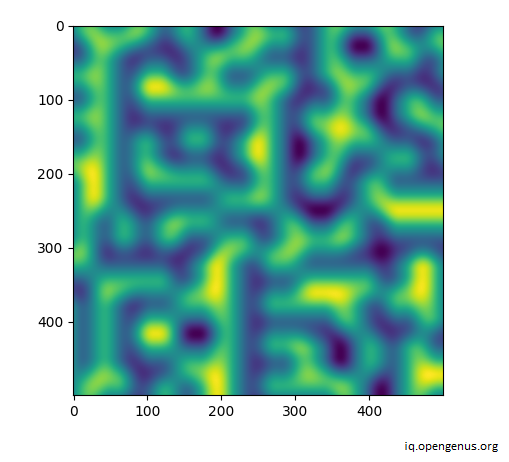# Complexity

Creating a Perlin image with 2 dimensions requires 4 gradient points. So, creating an image with n dimensions would require 2n points. Calculation happens at each of these points leading to a space requirement and time complexity of O(2n) in the best and worst case.

# Applications

• Computer Generated Imagery (CGI) makes extensive use of textures generated with the Perlin algorithm.

• Many nature-like computer-generated textures like fire, clouds, and smoke make use of the Perlin algorithm for a textural generation to mimic the randomness in natural phenomena.

• Perlin noise can generate textures with minimal memory requirement, and as such finds its use in many computer games and Graphics Processing Units (GPU).

With this article at OpenGenus, you must have the complete idea of Perlin Noise.